# Po-group

(diff) ← Older revision | Latest revision (diff) | Newer revision → (diff)
Jump to: navigation, search

partially ordered group

A group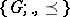endowed with a partial order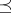such that for all,(Cf. also Partially ordered group.) Ifis the identity of a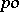-groupand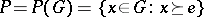is the positive cone of(cf.-group), then the following relations hold:

1)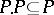;

2)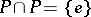;

3)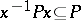for all.

If, in a group, one can find a setwith the properties 1)–3), thencan be made into a-group by setting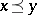if and only if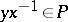. It is correct to identify the order of a-group with its positive cone. One often writes a-groupwith positive coneas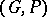.

A mapping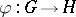from a-groupinto a-groupis an order homomorphism if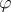is a homomorphism of the groupand for all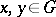,A homomorphismfrom a-groupinto a-group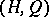is an order homomorphism if and only if.

A subgroupof a-groupis called convex (cf. Convex subgroup) if for allwith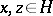,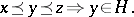Ifis a convex subgroup of a-group, then the setof right cosets ofbyis a partially ordered set with the induced order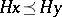if there exists ansuch that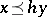. The quotient group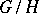of a-groupby a convex normal subgroupis a-group respect with the induced partial order, and the natural homomorphism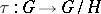is an order homomorphism. The homomorphism theorem holds for-groups: ifis an order homomorphism from a-groupinto a-group, then the kernel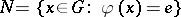ofis a convex normal subgroup ofand there exists an order isomorphismfrom the-group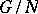intosuch that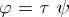.

The most important classes of-groups are the class of lattice-ordered groups (cf.-group) and the class of totally ordered groups (cf.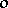-group).

This article extends and updates the article Partially ordered group (Volume 7).

How to Cite This Entry:
Po-group. Encyclopedia of Mathematics. URL: http://encyclopediaofmath.org/index.php?title=Po-group&oldid=11826
This article was adapted from an original article by V.M. Kopytov (originator), which appeared in Encyclopedia of Mathematics - ISBN 1402006098. See original article